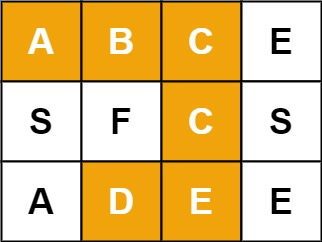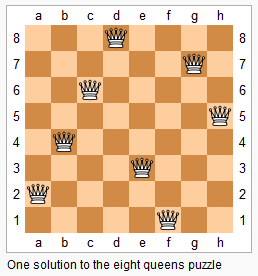# 79. 单词搜索

Given an m x n grid of characters board and a string word, return true if word exists in the grid.# DFS&BFS - 37. Sudoku Solver

Write a program to solve a Sudoku puzzle by filling the empty cells.# DFS - 980. Unique Paths III

980. Unique Paths III On a 2-dimensional grid, there are 4 types of squares: 1 represents the starting square.  There is exactly one starting square. 2 represents the ending square.  There is exactly one ending square. 0 represents empty squares we can walk over. -1 represents obstacles that we cannot walk over. Return the number of 4-directional walks from the starting square to the ending square, that walk over every non-obstacle square ....# DFS&BFS - 52. N-Queens II

52. N-Queens II The n-queens puzzle is the problem of placing n queens on an n_×_n chessboard such that no two queens attack each other. Given an integer n, return the number of distinct solutions to the n-queens puzzle. Example: Input: 4 Output: 2 Explanation: There are two distinct solutions to the 4-queens puzzle as shown below. [  [".Q..",  // Solution 1   "...Q",   "Q...",   "..Q."],  ["..Q.",  // Solution 2 &n....# DFS&BFS - 51. N-Queens

The n-queens puzzle is the problem of placing n queens on an n_×_n chessboard such that no two queens attack each other.# maze - dfs# DFS&BFS - 130. Surrounded Regions

130. Surrounded Regions Given a 2D board containing 'X' and 'O' (the letter O), capture all regions surrounded by 'X'. A region is captured by flipping all 'O's into 'X's in that surrounded region. Example: X X X X X O O X X X O X X O X X After running your function, the board should be: X X X X X X X X X X X X X O X X Explanation: Surrounded regions shouldn’t be on the border, which means that any 'O' on the border of the board are not flipped....# DFS&BFS - 200. Number of Islands

200. Number of Islands Given a 2d grid map of '1's (land) and '0's (water), count the number of islands. An island is surrounded by water and is formed by connecting adjacent lands horizontally or vertically. You may assume all four edges of the grid are all surrounded by water. Example 1: Input: 11110 11010 11000 00000 Output: 1 Example 2: Input: 11000 11000 00100 00011 Output: 3 思路： 题目意思是在一个二维平面找出有多少个岛屿，可以使用dfs或者bfs做，这里使用dfs来做，每找到一个点是岛屿，就不断四个方向探索，把探索过的区域打上标记或者置为水域就可以。 代....

Nothing just happens, it's all part of a plan.